### Visualising Solid Shapes - Solutions 2

CBSE Class –VII Mathematics
NCERT Solutions
Chapter 15 Visualising Solid Shapes (Ex. 15.2)

Question 1. Use isometric dot paper and make an isometric sketch for each one of the given shapes: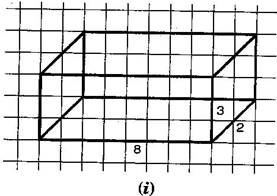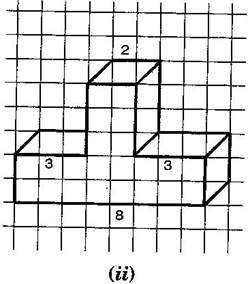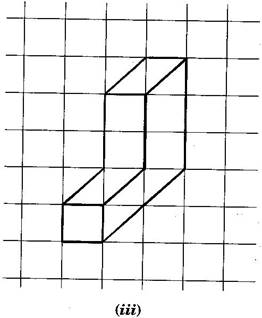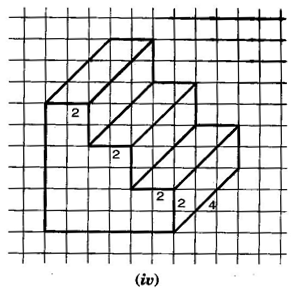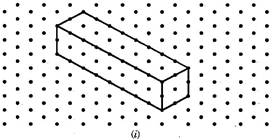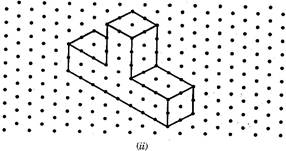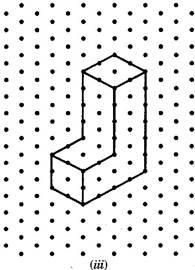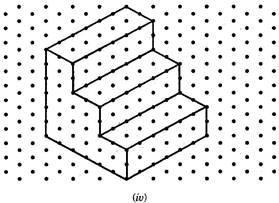Question 2. The dimensions of a cuboid are 5 cm, 3 cm and 2 cm. Draw three different isometric sketches of this cuboid.
Answers : The dimensions of given cuboid are 5 cm, 3 cm and 2 cm: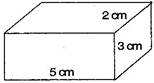Three different isometric sketches are: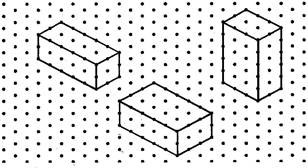Question 3. Three cubes each with 2 cm edge are placed side by side to form a cuboid. Sketch an oblique or isometric sketch of this cuboid.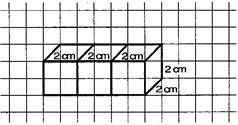Isometric sketch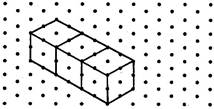Question 4. Make an oblique sketch for each one of the given isometric shapes: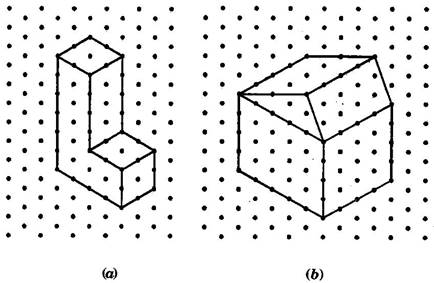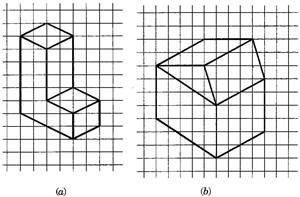Questions 5. Give (i) an oblique sketch and (ii) an isometric sketch for each of the following:
(a) A cuboid of dimensions 5 cm, 3 cm and 2 cm. (Is your sketch unique?)
(b) A cube with an edge 4 cm long.
Answers : (a) A cuboid of dimension 5 cm, 3 cm and 2 cm.
(i) Oblique sketch (ii) Isometric sketch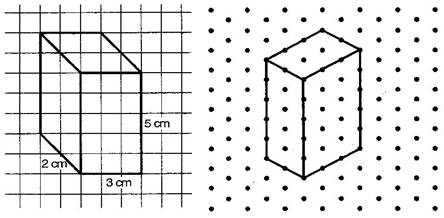(b) A cube with an edge 4 cm long.
(i) Oblique sketch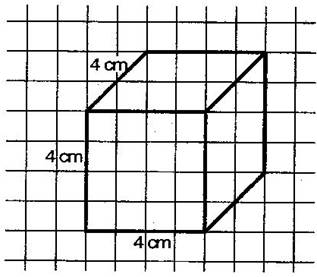(ii) Isometric sketch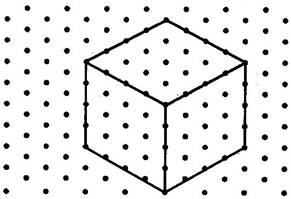Question 6. An isometric sheet is attached at the end of the book. You could try to make on it some cubes or cuboids of dimensions specified by your friend.
Answer : Cubes and cuboids shapes on isometric sheet given below: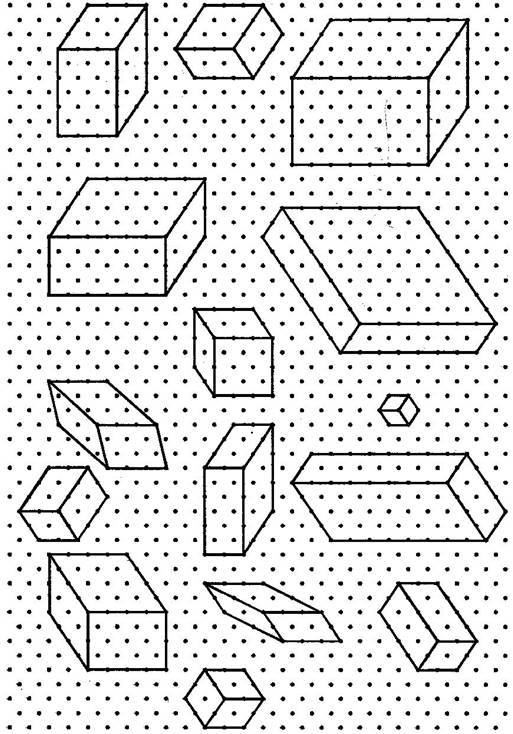You can also draw more shapes of cubes and cuboids.